ISEE Lower Level Quantitative : How to find the distributive property

Example Questions

2 Next →

Example Question #11 : How To Find The Distributive Property

Use the distributive property to solve for.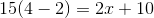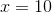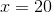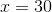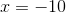Explanation:

The distributive property is needed to evaluate this expression.

The distributive property is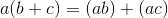Applying this property to each term within the parentheses we get the following.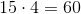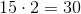Substituting these new values into our original expression we get: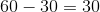Thus,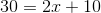Subtracting 10 from both sides,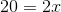therefore our final solution is,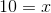Example Question #12 : How To Find The Distributive Property

Use distributive property to expand the expression.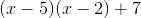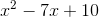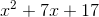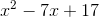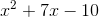Explanation:

The distributive property is needed to solve this problem. The distributive property is. In this particular, case the FOIL technique should be used to expand this expression. FOIL is an acronym that helps students remember to multiply the first terms in each parentheses, then the outside terms in each parentheses, followed by multiplying the inside terms and then finally multiplying the last terms in each parentheses.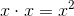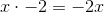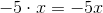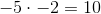The last step is to combine like terms. Thus, the correct answer is:Example Question #13 : How To Find The Distributive Property

Use the distributive property to solve for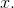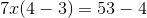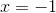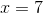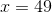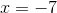Explanation:

The distributive property is needed to solve this problem.

The distributive property is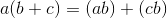Applying this property to each of the terms within the parentheses we get the following.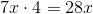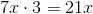Substituting these values back into the original expression we get,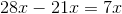on the left hand side and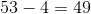on the right hand side.

Thus,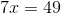Example Question #14 : How To Find The Distributive Property

Use the distributive property to find an equivalent expression.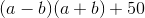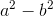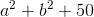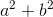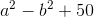Explanation:

The distributive property is needed to solve this problem. The distributive property is. In this particular, case the FOIL technique should be used to expand this expression. FOIL is an acronym that helps students remember to multiply the first terms in each parentheses, then the outside terms in each parentheses, followed by multiplying the inside terms and then finally multiplying the last terms in each parentheses.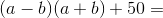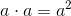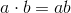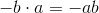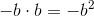The final step is to combine like terms. Thus, the correct answer is:Example Question #15 : How To Find The Distributive Property

Use the distributive property to solve for.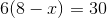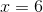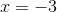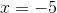Explanation:

The distributive property needs to be used to solve this expression for x.

The distributive property is:From here we need to subtract 48 from both sides to isolate -6x.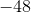Now we need to divide by -6 in order to solve for x.2 Next →